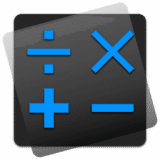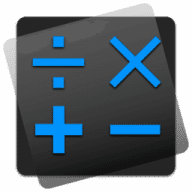Simple Arithmetics
1.4.5

0.0

Simple Arithmetics# Simple Arithmetics1.4.5

31 July 2017

Practice simple arithmetic.

## Overview

With Simple Arithmetics, you can practice addition, subtraction, multiplication, and division problems in the range from 0 to 400.

Enter the missing operators by pressing the operator buttons. The application tells you if the equations are correct. After finishing, a test a message appears that shows you how many points you scored.

Localisations: English, German

## What's new in Simple Arithmetics

Version 1.4.5:

Note: Now requires OS X 10.9 or later running on a 64-bit Intel processor.

• Minor UI changes
• Bug fixes

#### Join over 500,000 subscribers.

Subscribe for our newsletter with best Mac offers from MacUpdate.

How would you rate Simple Arithmetics app?